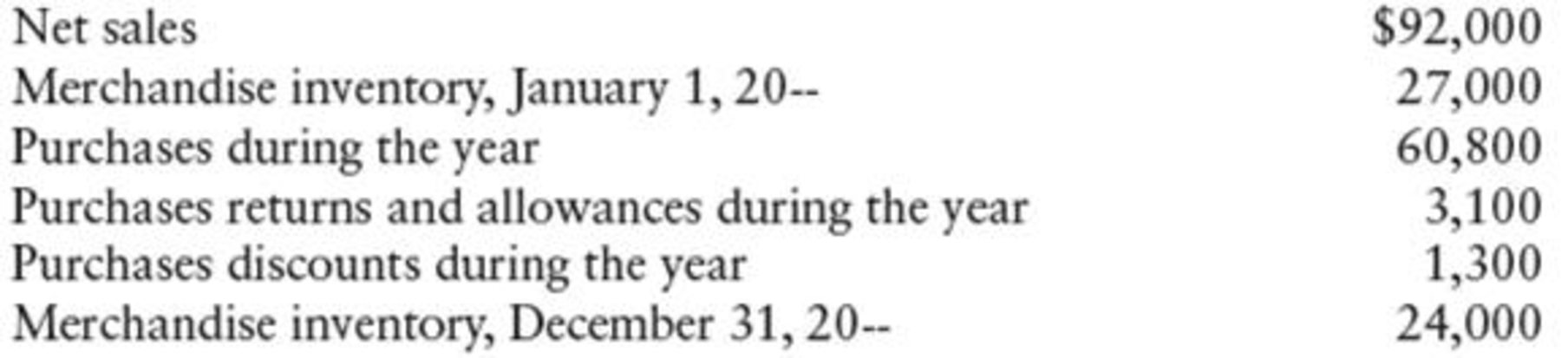Chapter 11, Problem 2CE

Chapter
Section
Textbook Problem

Based on the following data for Dave’s Supply House, determine the gross profit.To determine

Ascertain gross profit for S House.

Explanation

Gross profit: The excess of price of merchandise sold over the cost of merchandise sold is referred to as gross profit.

Formula to compute gross profit:

Gross profit = Net sales –�

Still sussing out bartleby?

Check out a sample textbook solution.

See a sample solution

The Solution to Your Study Problems

Bartleby provides explanations to thousands of textbook problems written by our experts, many with advanced degrees!

Get Started

Find more solutions based on key concepts